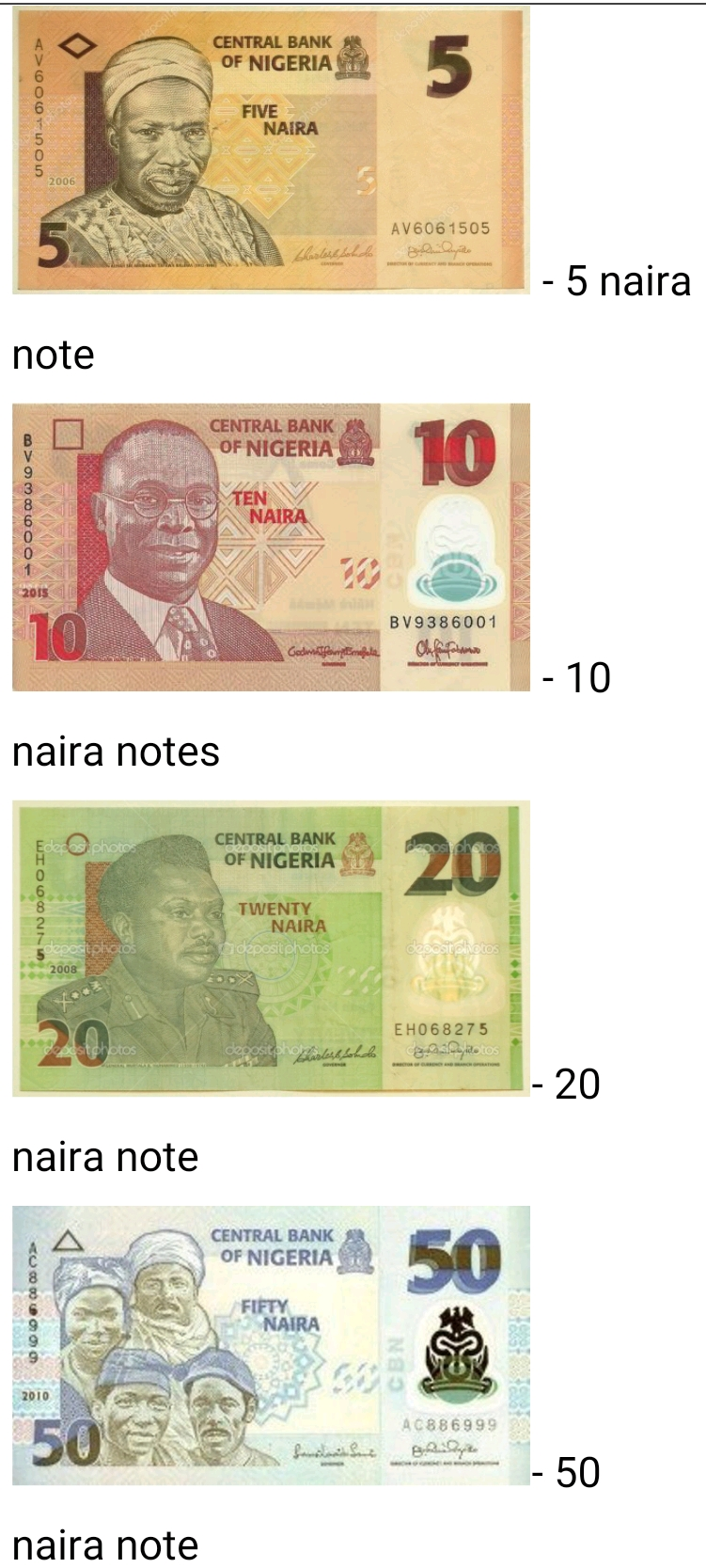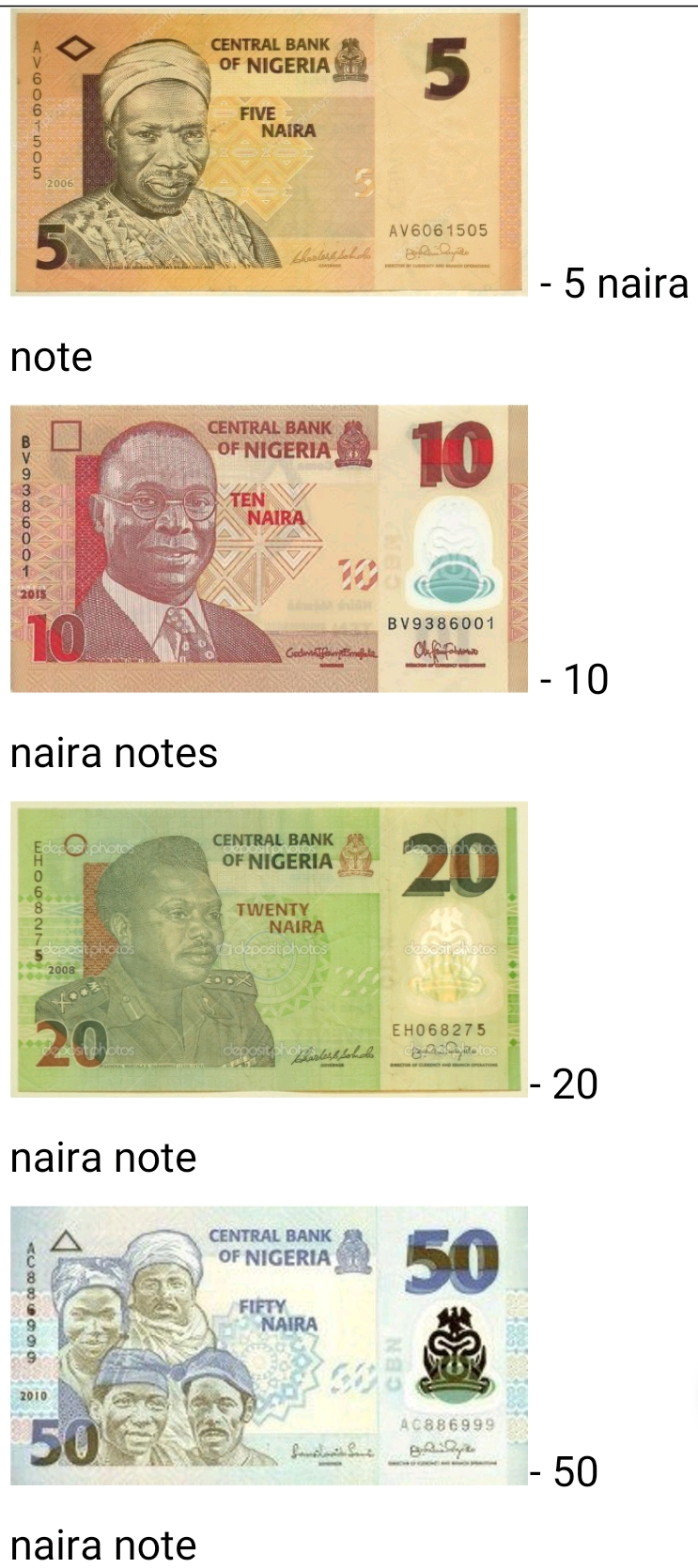# Lesson Notes By Weeks and Term - Nursery 2

Counting/identification of numbers 1 - 750

SUBJECT: Numeracy

TERM: 3rd Term

Week: 5

CLASS – Nursery 2

AGE – 5 years

DURATION – 5 periods of 35 minutes each

TOPIC- Counting/identification of numbers 1-750

CONTENT – Writing 450-500, Number endings. Number spellings 1-100. 10x table. fractions, Money problems

INSTRUCTIONAL OBJECTIVES – By the end of the lesson, the pupils should be able to –

1. Count and identify numbers 1-750
2. Write numbers 450-500
3. Write in words 1-100
4. Recite the 10x table
6. Tell the time
7. Identify all the naira notes

INSTRUCTIONAL MATERIALS – flash cards, charts, counters

INSTRUCTIONAL TECHNIQUES – Explanation, repetition, questions and answers

ENTRY BEHAVIOUR – The pupils can count numbers 1-700 and spell numbers 1-90

SET INDUCTION –She uses number rhyme to arouse their interest

INSTRUCTIONAL PROCEDURE

PERIOD I: Counting numbers 1-750 and writing 450-500

 STEPS TEACHER’S ACTIVITY PUPILS’ ACTIVITY IIntroduction The teacher reviews the previous lesson introduces the new topic Pupils participate IICounting Using the number chart, she counts number 1-750 repeatedly. Pupils repeat after her IIIWriting numbers She counts and write numbers 450-500 on the board; she also uses flashcards Pupils count and write in their book IVNumber ending She writes the number endings on the board and also uses flashcards Pupils count Evaluation Count and write numbers 450-500 Conclusion She marks their books and corrects them where necessary

PERIOD II& III: Number spellings 1-100. Addition of fractions, 10x times table

 STEPS TEACHER’S ACTIVITY PUPILS’ ACTIVITY IIntroduction The teacher reviews the previous lesson introduces the new topic Pupils participate IIDrilling She drills them on the spelling of 91-100Ninety-one = 91Ninety-two = 92Ninety-three = 93Ninety-four = 94Ninety-five = 95Ninety-six = 96Ninety-seven = 97Ninety-eight = 98Ninety-nine = 99One hundred = 100 IIIAddition of fractions She draws some fractions on the board and explains how to add themFor examples;Pupils identify heavy and light objects III10x table She writes the 10x table on the board and recites it severally as the pupils recite after her10 x 1 = 1010 x 2 = 2010 x 3 = 3010 x 4 = 4010 x 5 = 5010 x 6 = 6010 x 7 = 7010 x 8 = 8010 x 9 = 9010 x 10 = 10010 x 11 = 12010 x 12 = 140 Evaluation 1. Add these fractions2. Write in words 91-10091 =92 =93 =94 =95 =96 =97 =98 =99 =100 =3. Complete the 10x table10 x 1 =10 x 2 =10 x 3=10 x 4= Conclusion She marks their books and corrects them where necessary

PERIOD V: Identification of money

 STEPS TEACHER’S ACTIVITY PUPILS’ ACTIVITY IIntroduction The teacher reviews the basic shapes and introduces the new topic Pupils respond IIIntroduction to money The teacher displays all the currency notes and identify each one of themPupils pay attention Evaluation 1. Identify these naira notesConclusion She marks their books and corrects them where necessary